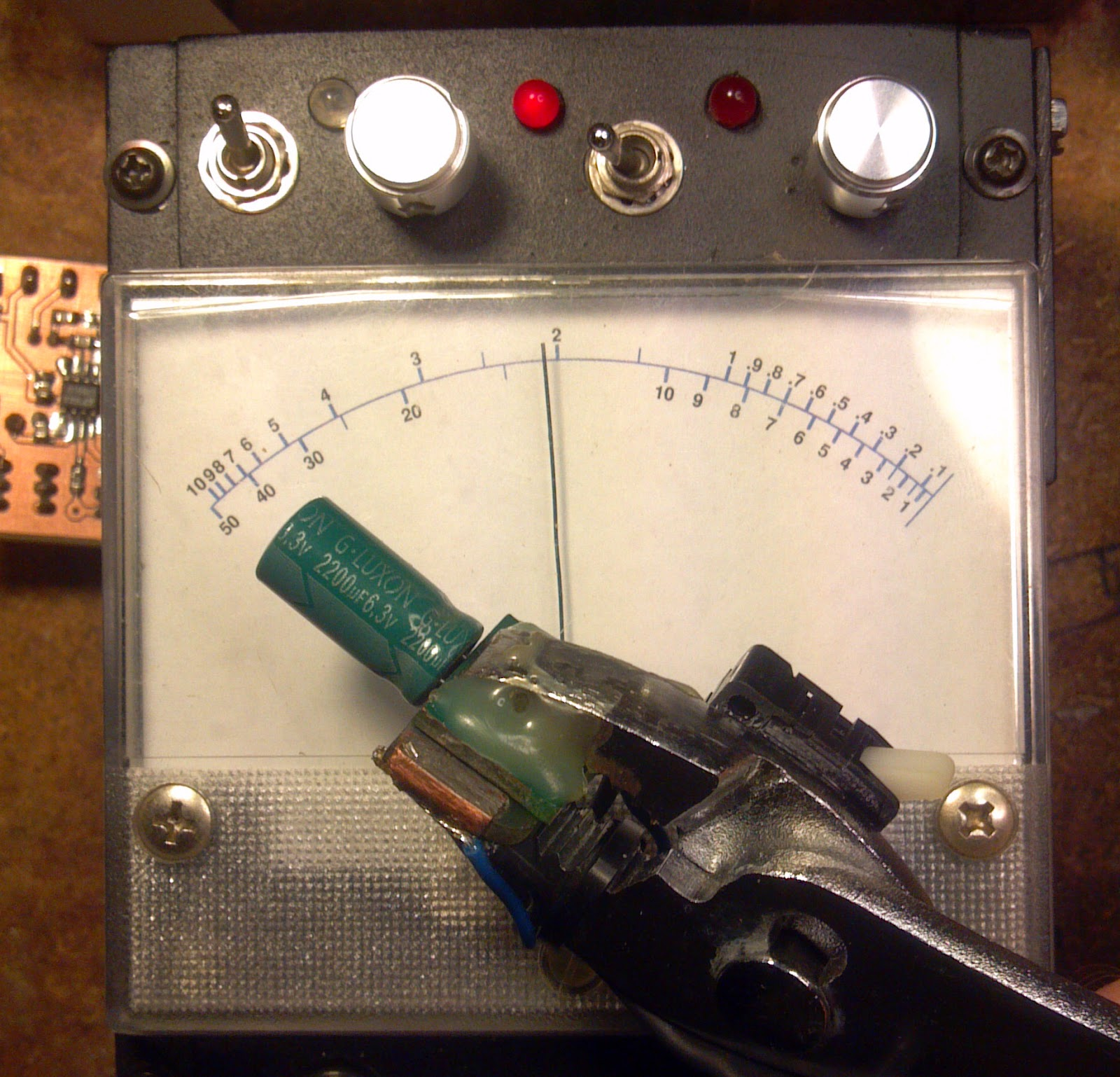## Sunday, March 29, 2015

### Create accurate custom meter legends

My ability to give a shit has worn abnormally thin lately; bear with me.  Maybe if I gain some reason to believe that this entire blog is anything more than a masturbatory distraction from an interminable hopelessness, I might bother to put some effort into things.

Analog meters are handy for making things with.  Stick it on an amplifier project or an ugly cobbled-together kludge you like to pretend is a remotely useful bit of test gear.  It doesn't matter where you use them; sometimes it's just easier to use an analog meter than a digital one.  The only real obstacle in any application is creating the corresponding legend for the meter face.  Here, I'll show my method which works well for nonlinear scales.With original meter face
In the course of finishing up my twice-aborted variant of Manfred Mornhinweg's ESR meter (I should've started from scratch), I decided that using a marker and some masking tape was just too ghetto for my tastes.  The scales in this application are not linear and I ended up needing dual scales to match the two range settings required in order to have a broad range and sufficient resolution for low resistance readings.

To start, I took a set of known resistors in the meter's range and correlated their values to the existing 0-16 linear scale.  I performed this test in both ranges, resulting in two sets of resistances and scale values.  I then measured the radius and chord length of the existing meter scale.Measure this shit so you can find section angle and legend size
Using MATLAB, the measurements are interpolated onto a new semi-logarithmic resistance space to yield a legend with convenient divisions.  Depending on the application, curve fitting, linear interpolation, or spline interpolation can be used.  I decided to just use a spline with some endslope definitions to taste.

```%% ESR meter scales
clc; clf; clear all;
format compact;

rtlo=[0.18 1 1.5 3 6.8 10 25];
rthi=[0.18 1 1.5 3 6.8 10 25 46];
xlo=[15.2 11.2 9.4 5 1 0.2 0];
xhi=[16 15.8 15.6 14.8 12.6 10 3.2 0.4];

% create semi logarithmic resistance space
%z = -1:1; d=1:9;
%R=cell2mat(arrayfun(@(i) d.*10^z(i),1:length(z),'UniformOutput',false));
R=[0 0.1 0.2 0.3 0.4 0.5 0.6 0.7 0.8 0.9 ...
1 1.5 2 2.5 3 4 5 6 7 8 9 ...
10 15 20 25 30 40 50];

% linear interpolation
%Xlo=interp1(rtlo,xlo,R);
%Xhi=interp1(rthi,xhi,R);

% or calculate from rational fit (or other function type; try cftool)
%fitlo=[-12.57 162.3 2.023 10.16];
%fithi=[-103.5 6662 -4.942e+04 -8.055 355.9 -3069];
%Xlo=(fitlo(1)*R + fitlo(2)) ./ (R.^2 + fitlo(3)*R + fitlo(4));
%Xhi=(fithi(1)*R.^2 + fithi(2)*R + fithi(3)) ./ (R.^3 + fithi(4)*R.^2 + fithi(5)*R + fithi(6));

% or use splines see if i care
Xlo=spline(rtlo,xlo,R);
Xhi=spline(rthi,[-0.15 xhi -0.10],R);

figure(1);
semilogx(rtlo,xlo,'r',R,Xlo,'c'); hold on; grid on;
semilogx(rthi,xhi,'r',R,Xhi,'c');
```Spline and original data (scale value versus resistance value)
The next step is to use plot functions to create a vector image that can be used as the final meter face. A sane person might invest this programmatic effort into directly assembling an SVG or something, but I'm just going to do it this way.
```%% curved scales
clf;
figure(2);
r=52; % scale centerline radius in mm
l=76; % scale chord length in mm
sw=2*acos(l/(2*r)); % scale swing in rad
fontsize=18;
linescale=0.5;

plot(,,'+');

axis([-40 40 0 60]);
axis equal;
for n=1:22;
if Xlo(n)<=16;
phi=sw/2 - (Xlo(n)/16)*sw + pi/2;
if sum(find(R(n)==[1.5 2.5 15 25]))==0;
offset=0.005*fontsize/10;
textstring=num2str(R(n));
if R(n) < 1;
else
end
set(t,'Rotation',(phi*180/pi)-90);
set(t,'FontWeight','bold');
set(t,'FontSize',fontsize);
end
if sum(find(R(n)==[1.5 2.5 15 25]))==0;
set(h,'LineWidth',2*linescale)
else
set(h,'LineWidth',1*linescale)
end
end
end

for n=1:length(Xhi);
if Xhi(n)<=16 && R(n)>=1;
phi=sw/2 - (Xhi(n)/16)*sw + pi/2;
if sum(find(R(n)==[1.5 2.5 15 25]))==0;
offset=0.01*fontsize/10;
set(t,'Rotation',(phi*180/pi)-90);
set(t,'FontWeight','bold');
set(t,'FontSize',fontsize);
end
if sum(find(R(n)==[1.5 2.5 15 25]))==0;
set(h,'LineWidth',2*linescale)
else
set(h,'LineWidth',1*linescale)
end
end
end

th=pi/2-sw/2:sw/100:pi/2+sw/2;
h=line(r*cos(th),r*sin(th));
set(h,'LineWidth',3*linescale)

set(h,'LineWidth',1*linescale)
```The scale as produced by the script using plot/draw tools
The font size and line weights can be set.  Number alignment can be adjusted for each scale using an offset factor.  If at this point, the results look a little off here or there, that's okay.  Once things are exported, they can be adjusted. Save the plot as a .eps file and throw it in Inkscape to adjust a few things that don't line up and then scale the output for the physical world.The scale in Inkscape after adjusting a few things
Proper print scaling should result in the X-Y scales on the original plot coming out such that they read in millimeters on the page.  A simpler way to ensure the scale size is correct is to simply print the thing and measure it to back-calculate a scaling correction factor.  Reprint the legend with the correction factor and use the original meter scales to help with positioning. Cut it to size and mount it with spray adhesive.  Turn off the lights and stop caring.Measuring a bad LUXON capacitor with the new meter face
This beats trying to eyeball things anyway.  Maybe someday I'll write about the ESR meter and the changes I made to the original design.  Maybe someday I'll have better things to do.  Maybe someday unicorns will fly out of my ass.

No wait.  Not unicorns.  That would be bad.